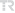## Fixed-point theorems in extended fuzzy metric spaces via \$alpha-phi-mathcal{M}^{0}\$ and \$beta-psi-mathcal{M}^{0}\$ fuzzy contractive mappings

Communications Faculty of Sciences University of Ankara Series A1: Mathematics and Statistics, vol.72, no.1, pp.71-83, 2023 (ESCI)#### Abstract

In this article we would like to present a new type of fuzzy contractive mappings which are called \$alpha-phi-mathcal{M}^{0}\$ fuzzy contractive and \$beta-psi-mathcal{M}^{0}\$ fuzzy contractive, and then we demonstrate two theorems which ensure the existence of a fixed point for these two types of mappings. And so we combine and generalize some existing notions in the literature (, ). Proved these theorems in the extended fuzzy metric spaces are in the more general version than the existing in the literature ones.